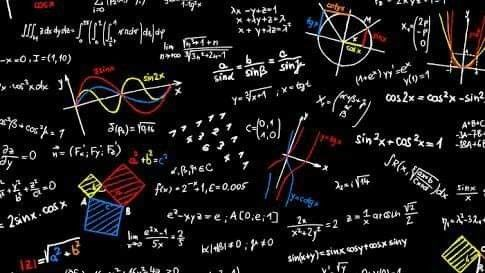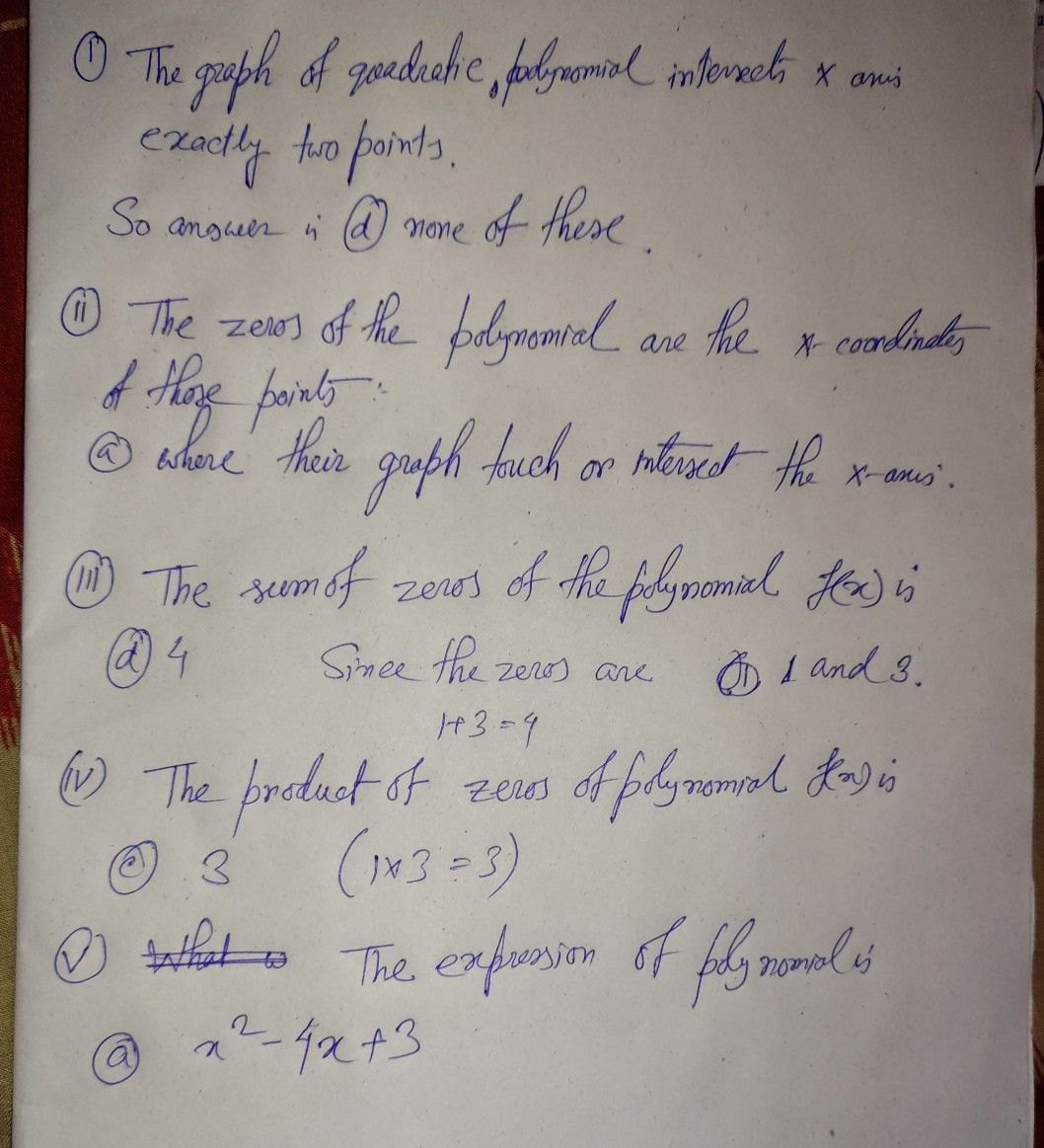Symbol
Problem$Q^{19}$ A student was given the task to prepare a graph of quadratic polynomial $f\left(x\right).T0$ draw this graph he take several values of y corresponding to different values of x. After plotting the points on the graph paper with suitable $sca|e$ he obtain the graph as shown below $y=f\left(x\right)$ 43- - 2+ 1(0, 1) 0) $30$ $\vec{4} ^{x}$ $x\vec{+-4}$ (1, -14 -2+ Based on the above graph, answer the following $9uestions$ (i) The graph of quadratic polynomial intersects $x-a\times i5$ : (a) atleast at two points (b) atmost at two points (c) exactly one point (d) none of these. (ii) The zeroes of the polynomial are the $x-coordinates$ of those points: (a) where their graph touch or intersect the $x-axis$ (b) where their graph touch or intersect the $y-axi5$ Scanned by T (c) where their graph touch or intersect both axes. (iii) T(dh) e none of these. sum of zeroes of the polynomial $f\left(x\right)is$ (a) 1 (b) 2 3 $\left(d\right)4$ (iv) T(hc) e product of zeroes of the polynomial $f\left(x\right)lsi$ $\left(b\right)2$ $\left(d\right)$ $4$ $omia1$ (v) AW(((acaXh) ) ) )a? 3 1 t will be the expression of Polynomial - $x^{2}-4x+3$ $x^{2}+4x-3$ (b) $x^{2}-3x+4$ $x^{2}+3x-4$ (c) (d)
10th-13th grade
Other
Search count: 109
SolutionQanda teacher - StudyPointIf you have any doubt about this question ask me. otherwise Please evaluate the answer. If you are satisfied give 5 star rating . If you want you may give thank you gift